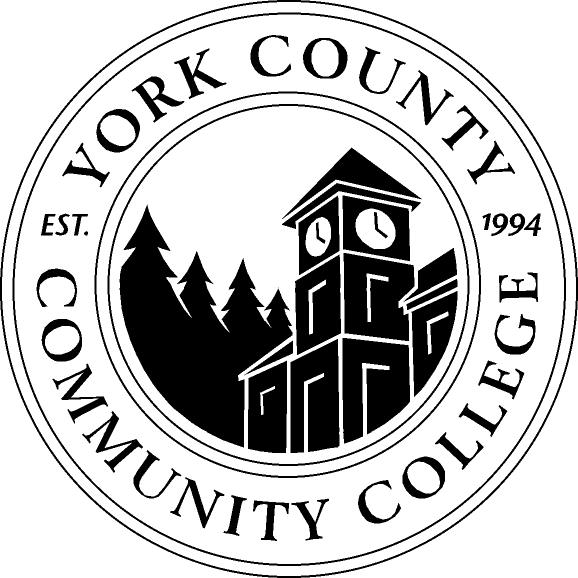It looks like you're using Internet Explorer 11 or older. This website works best with modern browsers such as the latest versions of Chrome, Firefox, Safari, and Edge. If you continue with this browser, you may see unexpected results.

# Accuplacer Prep: Accuplacer Prep Program

## Accuplacer PrepPrep for the Accuplacer at YCCC           Offering students the opportunity to prepare, place and progress!

## Video Tutorials by Topic from Khan Academy

What is the Accuplacer?

York County Community College uses the Accuplacer to determine the most appropriate placement into math and english courses.  It is a multiple choice placement test that focuses on the following:

English Placement (2 parts):

Sentence Skills

The Sentence Skills test, comprised of 20 questions, measures your understanding of sentence structure — what makes a sentence complete and clear. Some questions deal with the logic of the sentence, and others with the relationships between two sentences.

The Reading Comprehension test, comprised of 20 questions, measures your ability to understand what you read, to identify main ideas and to make inferences. You need to distinguish between direct statements and secondary or supporting ideas.

Arithmetic is associated with MAT-092, Elementary is associated with MAT-098 and College Algebra is associated with college level math classes at YCCC.

Arithmetic

The Arithmetic test, comprised of 17 questions, measures your ability to perform basic arithmetic operations and to solve problems that involve fundamental arithmetic concepts. There are three types of Arithmetic questions:

• Operations with whole numbers and fractions: topics included in this category are addition, subtraction, multiplication, division, recognizing equivalent fractions and mixed numbers, and estimating.
• Operations with decimals and percents: topics include addition, subtraction, multiplication, and division with decimals. Percent problems, recognition of decimals, fraction and percent equivalencies, and problems involving estimation are also given.
• Applications and problem solving: topics include rate, percent, and measurement problems, simple geometry problems, and distribution of a quantity into its fractional parts.

Elementary Algebra

The Elementary Algebra test, comprised of 12 questions, measures your ability to perform basic algebraic operations and to solve problems involving elementary algebraic concepts. There are three types of Elementary Algebra questions:

• Operations with integers and rational numbers: topics include computation with integers and negative rationals, the use of absolute values, and ordering.
• Operations with algebraic expressions: topics include the evaluation of simple formulas and expressions, adding and subtracting monomials and polynomials, multiplying and dividing monomials and polynomials, the evaluation of positive rational roots and exponents, simplifying algebraic fractions, and factoring.
• Solution of equations, inequalities, word problems: topics include solving linear equations and inequalities, solving quadratic equations by factoring, solving verbal problems presented in an algebraic context, including geometric reasoning and graphing, and the translation of written phrases into algebraic expressions.

College Level Math

The College-Level Math test, comprised of 20 questions, measures your ability to solve problems that involve college-level mathematics concepts. There are five types of College-Level Math questions:

• Algebraic operations: topics include simplifying rational algebraic expressions, factoring, expanding polynomials, and manipulating roots and exponents.
• Solutions of equations and inequalities: topics include the solution of linear and quadratic equations and inequalities, equation systems and other algebraic equations.
• Coordinate geometry: topics include plane geometry, the coordinate plane, straight lines, conics, sets of points in the plane, and graphs of algebraic functions.
• Applications and other algebra topics: topics include complex numbers, series and sequences, determinants, permutations and combinations, fractions and word problems.
• Functions and trigonometry: topics include polynomials, algebraic, exponential, and logarithmic and trigonometric functions.

## Resources for Purchase### The Official ACCUPLACER iPhone App

The Official ACCUPLACER Study App is intended to familiarize you with the basic content areas of the ACCUPLACER test. The app provides interactive sample questions on the following ACCUPLACER tests: Arithmetic, Elementary Algebra, College-Level Math, Reading Comprehension and Sentence Skills. With this app you can:

• access interactive sample questions on all ACCUPLACER tests
• get immediate and detailed feedback on correct or incorrect answers

The app is compatible with iPhone, iPod touch and iPad, and requires iOS 4.2 or later.

### The Official Web-Based Study App

The web-based study app features practice tests in Arithmetic, Elementary Algebra, College-Level Math, Reading Comprehension and Sentence Skills. You can access the app at any computer and familiarize yourself with the basic content areas of ACCUPLACER tests. With this app, you can enjoy the following features:

• “Learn as you go” tests provide you with explanation of the correct or incorrect responses
• Sample tests help you experience what a real test may be like on test day
• Save your work and process, and resume practicing at a later time
• Review your score history to identify areas of strength and weaknesses

## Take the Accuplacer Again

If you haven't taken a math class at YCCC and you would like to take the Accuplacer again, please contact the Admissions Department at 646-9282 to schedule a time.